# DPASF: a flink library for streaming data preprocessing

## Abstract

### Background

Data preprocessing techniques are devoted to correcting or alleviating errors in data. Discretization and feature selection are two of the most extended data preprocessing techniques. Although we can find many proposals for static Big Data preprocessing, there is little research devoted to the continuous Big Data problem. Apache Flink is a recent and novel Big Data framework, following the MapReduce paradigm, focused on distributed stream and batch data processing.

In this paper, we propose a data stream library for Big Data preprocessing, named DPASF, under Apache Flink. The library is composed of six of the most popular and widely used data preprocessing algorithms. It contains three algorithms for discretization, and three algorithms for performing feature selection.

### Results

The algorithms have been tested using two Big Data datasets. Experimental results show that preprocessing can not only reduce the size of the data, but also maintain or even improve the original accuracy in a short period of time.

### Conclusion

DPASF contains algorithms that are useful when dealing with Big Data data streams. The preprocessing algorithms included in the library are able to tackle Big Datasets efficiently and to correct imperfections in the data.

## Background

In recent years, the amount of data generated can no longer be treated directly by humans or manual applications, there is a need to analyze this data automatically and on a large scale . This is what is know to be Big Data, data generated at high volume, velocity and variety. This kind of data requires a new high-performance processing and it can currently be found in many different fields .

In order to extract quality information from data, a previous step to learning must be performed. This is known as data preprocessing , this step is almost mandatory in order to obtain a good model. Data preprocessing  deals with missing values, noise, and redundant features [1, 5] among other factors.

Data streams  are sequences of unbounded and ordered data that arrive one at a time. This imposes restrictions on the learning algorithms which do not appear in static data. Therefore, new algorithms that can deal with this kind of data must be developed.

To address the problem of dealing with such a large amount of data in the Big Data era, distributed frameworks such as Apache Spark  and Apache Flink [8, 9] have been developed. Apache Spark is well known and designed to be a fast and general engine for large-scale in-memory data processing. Apache Flink focuses, on the other hand, on distributed streams and batch data processing . Flink is also the only system to incorporate a distributed dataflow runtime that exploits streaming pipelined executions for both stream and batch data processing. It also provides a fault tolerance mechanism, using a lightweight checkpoint system, which ensures that every data example will be reflected exactly once .

This paper presents the data stream library for Big Data preprocessing named DPASF, where six data preprocessing algorithms are implemented for Apache Flink, focusing on the discretization and feature selection problems. The algorithms chosen are those that have obtained the best performance in the survey carried out in . In this survey authors summarize, categorize and analyze the most relevant contributions on data preprocessing that cope with streaming data. The selected algorithms are InfoGain , FCBF  and OFS  for feature selection and IDA , LOFD  and PiD  for discretization.

The rest of the paper describes the theoretical background of the algorithms, experimental results and a brief tutorial on how to use them. Source code is available on GitHubFootnote 1 . The algorithms chosen are the most representative in data streaming preprocessing and, in addition, have shown positive results.

### Big Data

In general, Big Data  is known to be data that is too big or too complex to handle with conventional tools and with a single machine. For this reason, there is an increasing need to develop new tools that can handle all this amount of data efficiently. As a result, distributed frameworks like Hadoop , Spark  and Flink  were developed. These types of frameworks allow large amounts of data to be processed in a scalable way.

One common way to define Big Data is to describe it in terms of three dimensions, also known as the 3 V’s  (Volume, Velocity and Variety). Volume just refers to how much data there is. Velocity refers to the speed at which data is processed and analyzed. Lastly, Variety refers to how many different data formats there are to be analyzed.

### Data Streaming

The main characteristics of streaming data  are the following. In streaming data, instances are not available beforehand, they become available in a sequence fashion, one by one, or in batches. Instances can arrive quickly and at irregular time intervals. As streaming data is unbounded, it may be infinite and therefore cannot be stored in memory. Each instance is accessed usually only once and is then discarded. In order to provide real-time processing, instances are processed within a limited amount of time. The intrinsic characteristics of data are subject to change over time, this is what is know as concept drift .

Concept drift  is the main problem within streaming data, as it is important for the algorithm to detect it and update the learned model to reflect the underlying changes in the data.

### Data Preprocessing

Before applying any data mining process, it is necessary to adapt the data to the requirements imposed by each learning algorithm and clean the data properly.

Although data preprocessing is a critical step, it is often time consuming. There are two types of data preprocessing, those designed to reduce the complexity in the data, and those designed to prepare the data, this means data transformation, cleaning, normalizing etc. The former is called data reduction, the latter data preparation. When these techniques are applied, the data is in its final stage to be fed to the data mining algorithm.

Among data transformation, feature selection [1, 27] takes care of selecting only relevant and non-redundant attributes. The aim of this type of data preprocessing is to obtain a subset of the original data that stills maintains the ability to describe the inherent concept. As a side effect, reducing the complexity of the data also results in better efficiency in terms of the amounts of time to learn a model, as well as preventing over-fitting.

Discretization  is a technique that reduces the complexity of the data by dividing the domain of the variables into bins defined by cut points. This process transforms quantitative values into qualitative, as cut points define a set of non-overlapping intervals. Once the algorithm has computed cut points for each attribute, data is then mapped to its corresponding interval .

Although Apache Spark and Apache Flink may appear to be similar, they are designed to address different problems. Apache Spark process all data using a batch approach, it lacks a true stream processing. Apache Flink fills this gap. Flink provides both kinds of processing, batch and streaming, except Flink process streaming data as it happens, in an online fashion. In other words, Spark "emulates" streaming by processing streaming data in mini-batches, whereas Flink process them online. This makes Flink more efficient in terms of low latency.

Apache Flink has a fault tolerance system in order to recover from exceptions that may occur. It is designed to work at low latency even with large amounts of data.

## Theoretical description of the algorithms presented

This section presents a theoretical description of the implemented algorithms, as well as an introduction to feature selection (InfoGain , FCBF  and OFS ) and discretization (IDA , LOFD  and PiD ).

### Feature Selection

Feature selection  is meant to reduce the dimensionality of a dataset by removing irrelevant and redundant features. By doing this, a subset of the original features that still describes the inherent concept behind the data is returned. FS methods can be divided in the following categories:

• Wrapper Methods It uses an external evaluator, which depends on a learning algorithm.

• Filtering Methods It uses selection techniques based on separability measures or statistical dependencies.

• Embedded Methods It uses a search procedure implicitly embedded in the classifier or regressor.

In general, filtering methods tend to achieve better results when generalizing due to learning independence. In addition, filter methods are more efficient than wrapper methods, since the latter need to learn a model first. Therefore, in the context of big data, filtering methods are more widely used. Information Gain , OFS  and FCBF  are the most popular preprocessing algorithms in this area.

#### Information Gain

This feature selection scheme, described in  is composed of two steps: An incremental feature ranking method, and an incremental learning algorithm that can consider a subset of the features during prediction.

For this algorithm, the conditional entropy with respect to the class is computed with

$$H(X|Y) = -\sum_{j} P(y_{j})\sum_{i} P(x_{i}|y_{j})\log_{2}(P(x_{i}|y_{j}))$$
(1)

then, the Information Gain (IG) is computed for each attribute with

$$IG(X|Y) = H(X) - H(X|Y)$$
(2)

Once the algorithm has all Information Gain values for each attribute, the top N are selected as best features.

#### Online Feature Selection (OFS)

OFS  proposes an ε-greedy online feature selection method based on weights generated by an online classifier (neural networks) which makes a trade-off between exploration and exploitation of features.

The main idea behind this algorithm is that when a vector x falls withing a L1 ball, most of its numerical values are concentrated in its largest elements, therefore, removing the smallest values will result in a small change in the original vector x as measured by the Lq norm. This way, the classifier is restricted to a L1 ball:

$$\Delta_{R} = \left\{ \mathbf{w} \in {\sc{R}}^{d} : ||\mathbf{w}||_{1} \leq {\sc{R}} \right\}$$
(3)

OFS maintains an online classifier wt with at most B nonzero elements. When an instance (xt,yt) is incorrectly classified, the classifier gets updated through online gradient descent and then it is projected to a L2 ball to delimit the classifier norm. If the resulting classifier $$\hat {\mathbf {w}}_{t+1}$$ has more than B nonzero elements, the elements with the largest absolute value will be kept in $$\hat {\mathbf {w}}_{t+1}$$.

The above approach presents an inefficiency, even although the classifier consists of B nonzero elements, full knowledge of the instances is required, that is, each attribute xt must be measured and computed. As a solution, OFS limit online feature selection to no more than B attributes of xt

#### Fast Correlation-Based Filter (FCBF)

FCBF  is a multivariate feature selection method where the class relevance and the dependency between each feature pair are taken into account. Based on information theory, FCBF uses symmetrical uncertainty to calculate dependencies of features and the class relevance. Starting with the full feature set, FCBF heuristically applies a backward selection technique with a sequential search strategy to remove irrelevant and redundant features. The algorithm stops when there are no features left to eliminate.

The algorithm chooses as a correlation measure the entropy of a variable X, which is defined as

$$H(X) = -\sum_{i} P(x_{i})\log P(x_{i})$$
(4)

and the entropy of X after observing values of another variable Y is defined as

$$H(X|Y) = -\sum_{j} P(y_{j})\sum_{i} P(x_{i}|y_{j})\log_{2}(P(x_{i}|y_{j}))$$
(5)

where P(xi) is the prior probability for all values of X and P(xi|yj) is the posterior probability of X given the values of Y. With this information, a measure called Information Gain can be defined:

$$IG(X|Y) = H(X) - H(X|Y)$$
(6)

According to IG, a feature Y is more correlated to X than to a feature Z if IG(X|Y)>IG(Z|Y).

Now we are ready to define the main measure for FCBF, symmetrical uncertainty . As a pre-requisite, data must be normalized in order to be comparable.

$$SU(X, Y) = 2\left [\frac{IG(X|Y)}{H(X) + H(Y)} \right ]$$
(7)

SU compensate the bias in IG toward features with more values and normalizes its values to the range [0,1]. A SU value of 1 indicates total correlation whereas a value of 0 indicates independence.

The algorithm follows a two step approach, first, it has to decide if a feature is relevant to the class and two, decide if those features are redundant with respect to each other.

To solve the first step, a user defined SU threshold can be defined. If SUi,c is the SU value for feature Fi with the class c, the subset S of relevant features can be defined with a threshold δ such that FiS,1≤iN,SUi,cδ.

For the second step, in order to avoid analysis of pairwise correlations between all features, a method to decide whether the level of correlation between two features in S is high enough to produce redundancy is needed in order to remove one of them. Examining the value SUj,iFjS(ji) allows the level to which Fj is correlated by the rest of features in S to be estimated.

The last piece of the algorithm comprises two definitions:

### Definition 1

(Predominant Correlation). The correlation between a feature Fi and the class C is predominant iff SUi,cδ and $$\forall F_{j} \in S^{\prime } (j \neq i) \nexists F_{j}$$ such that SUj,iSUi,c

If such feature Fj exists for a feature Fi, it is called a redundant peer of Fi and it is added to a set $$S_{P_{i}}$$ identifying all the redundant peers for Fi. $$S_{P_{i}}$$ is divided into two parts: $$S_{P_{i}}^{+}$$ and $$S_{P_{i}}^{-}$$, where $$S_{P_{i}}^{+} = \{F_{j}|F_{j}\in S_{P_{i}}, {SU}_{j,c} > {SU}_{i,c}\}$$ and $$S_{P_{i}}^{-} = \{F_{j}|F_{j}\in S_{P_{i}}, {SU}_{j,c} \leq {SU}_{i,c}\}$$

### Definition 2

(Predominant Feature). A feature is predominant to the class if its correlation to the class is predominant or can become predominant after removing all its redundant peers.

According to the above definitions, a feature will be a good feature if it is predominant in predicting the class. These two definitions along with the following heuristics can effectively identify predominant features and remove the need of pairwise comparisons.

Heuristic 1 (when $$S_{P_{i}}^{+} = \emptyset$$). Fi is a predominant feature, delete all features in $$S_{P_{i}}^{-}$$ and stop searching for redundant peers for those features.

Heuristic 2 (when $$S_{P_{i}}^{+} \neq \emptyset$$). All features in $$S_{P_{i}}^{+}$$ are processed before making decisions in Fi. If none of them become predominant go to Heuristic 1, or else remove Fi and decide if features in $$S_{P_{i}}^{-}$$ need to be removed based on other features in S.

Heuristic 3 (Start point). The algorithm begins examining the feature with the largest SUi,c, as this feature is always predominant and acts as a starting point for the removal of redundant features.

### Discretization

Broadly speaking, discretization  translates quantitative data into qualitative data, trying to avoid an overlap between the continuous domain of the variable. This process results in a mapping of a value to a given interval. For this reason, discretization can be considered as a data reduction process, since it reduces data from a numerical domain to a subset of categorical values.

More formally, a discretization δ of a numeric attribute Xi is a set of m intervals called bins. The bins are defined by cut points {k1,…,km−1} that divide the domain of Xi into m bins where b1=[−,k1],bm=[km−1,] and for 1<i<m,bi=(ki−1,ki]. Therefore, a discretization for an attribute Xi is a mapping between values v of Xi and its bin indexes δv=z such that vbz.

The most popular discretization algorithms are IDA , PiD  and LOFD .

#### Incremental Discretization Algorithm (IDA)

IDA  approximates quantile-based discretization on the entire data stream encountered to date by maintaining a random sample of the data which is used to calculate the cut points. IDA uses the reservoir sampling algorithm to maintain a sample drawn uniformly at random from the entire stream up until the current time.

In IDA, a random sample is used because it’s not feasible for high-throughput streams to maintain a complete record of all the values seen so far. The sample method used is called reservoir sampling , and mantains a random sample of s values Vi for each attribute Xi. The first s values that arrive for each Xi are added to its corresponding Vi. Thereafter, every time a new instance 〈xn,yn〉 arrives, each of its values $$\mathbf {x}_{n}^{i}$$ replace a randomly selected value of the corresponding Vi with probability s/n.

Each value of each attribute is stored in a vector of interval heaps . $$V_{i}^{j}$$ stores the values for the j th bin of Xi. The reason to use a Interval Heap is that it provides efficient access to minimum and maximum values in the heap and direct access to random elements within the heap.

#### Partition Incremental Discretization algorithm (PiD)

PiD  performs incremental discretization. The basic idea is to perform the task in two layers. The first layer receives the sequence of input data and keeps some statistics on the data using many more intervals than required. Based on the statistics stored by the first layer, the second layer creates the final discretization. The proposed architecture processes streaming examples in a single scan, in constant time and space even for infinite sequences of examples.

PiD  performs incremental discretization. The basic idea is to perform the task in two layers.

The first layer receives the sequence of input data and the range of the variable and keeps some statistics on the data using many more intervals than required. The range of the variable is used to initialize the cut points with the same width. Each time a new value arrives, this layer is updated in order to compute the corresponding interval for the value. Each interval has a internal count of the values it has seen so far. When a counter for an interval reach a threshold, a split process is triggered to generate two new intervals. If the interval triggering the split process is the last or the first, a new interval with the same step is created. Otherwise the interval is split in two. In summary, the first layer simplifies and summarizes the data.

Based on the statistics stored by the first layer, the second layer creates the final discretization. The proposed architecture processes streaming examples in a single scan, in constant time and space even for infinite sequences of examples. To do so, this layer merges the set of intervals computed in the previous layer.

PiD stores the information about the number of examples per class in each interval in a matrix. In this matrix, columns correspond with the number of intervals and rows with the number of classes. With this information, the conditional probability of an attribute belonging to an interval given that the corresponding example belongs to a class can be computed as P(bi<xbi+1|Classj).

To perform the actual discretization Recursive entropy discretization  is used. This algorithm was developed by Fayyad and Irani . It uses the class information entropy of two candidate partitions to select the boundaries for discretization. It begins searching for a single threshold that minimizes the entropy over all possible cut points, then, it is applied recursively to both partitions. It uses the minimum description length  principle as stop criteria. The algorithm works as follows:

First, the entropy before and after the split is computed as well as its information gain. Then, the entropy for both left and right splits is computed and finally the algorithm checks if the split is accepted using the following formula

$$Gain(A,T;S) < \frac{\log_{2} (N-1)}{N} + \frac{\Delta (A, T;S)}{N}$$
(8)

where N is the number of instances in the set S,

$$Gain(A,T;S) = H(S) - H(A,T;S)$$
(9)

and

$$\Delta(A,T;S) = \log_{2} \left(3^{k} - 2\right) - \left [ k\cdot H(S) - k_{1} \cdot H(S_{1}) - k_{2} \cdot H(S_{2}) \right ]$$
(10)

where k i is the number of class labels represented in the set S i.

#### Local Online Fusion Discretizer (LOFD)

LOFD  is an online, self-adaptive discretizer for streaming classification. It smoothly adapts its interval limits reducing the negative impact of shifts and analyzes interval labeling and interaction problems in data streaming. Interaction discretizer-learner is addressed by providing two similar solutions. The algorithm generates an online and self-adaptive discretization solution for streaming classification which aims at reducing the negative impact of fluctuations in evolving intervals.

The algorithm is composed of two phases, the main process, at instance level, and the merge/split process, at interval level. The main process works as follows. First, discrete interval are initialized following the static process defined in . The discretization is then performed on the first initTh instances. From that moment on, LOFD updates the scheme of intervals in each iteration and for each attribute. For each new instance, it retrieves its ceiling interval (implemented as a red-black tree). If the point is above the upper limit a new interval is generated at that point, making that point the new maximum for the current attribute. A merge between the old and the new last interval is evaluated by computing the quadratic entropy, if the result is lower than the sum of both parts, the merge is accepted.

Finally, each point is added to a queue with a timestamp to control future removals in case the histogram overflows. If necessary, LOFD recovers points from the queue in ascending order and removes them until there is space left in the histogram.

The split/merge phase is triggered each time a boundary point is processed. The new boundary point splits an interval in two, one interval contains the points in the histogram with values less than or equal to the new point and keeps the same label. Each time a new interval is generated, the merge process is triggered for the intervals being divided and their neighbors.

## Implementation

This section presents the distributed design of the six implemented algorithms. For the implementation of the methods, we have used some basic Flink primitives. Here we outline those that are more relevant for the algorithms:

• map The Map transformation applies a user-defined map function to each element of a DataSet

• reduce A Reduce transformation reduces the dataset to a single element using a user-defined reduce function.

• mapPartition MapPartition transforms a parallel partition in a single function call.

• reduceGroup A GroupReduce transformation that is applied to a grouped DataSet calls a user-defined group-reduce function for each group. The difference between this and Reduce is that the user defined function gets the whole group at once.

Algorithm 1 shows pseudocode for FCBF, the SU value is computed for each attribute in parallel. All SU values are then filtered according to the threshold parameter and then sorted in descending order. With these final sorted values, the FCBF algorithm is applied as originally described in . Algorithm 2 shows how Symmetrical Uncertainty is computed in a distributed fashion. First, each parallel partition computes the partial counts of each value, then this partial counts are aggregated using a reduce function in order to compute the total counts. With this information, probabilities for each value are computed and the entropy and mutual information are calculated. Finally, it returns the corresponding SU value for that attribute.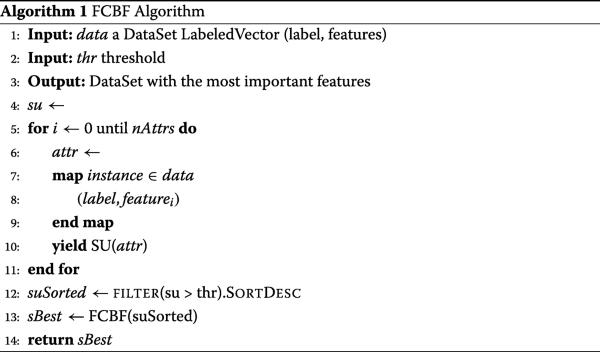Algorithm 3 shows the implementation for Information Gain . First the frequencies of each value with respect to the class label are computed. With this information, the total entropy of the dataset is computed. Next, for each attribute, its frequency, probability, entropy and conditional entropy are computed. Finally, the information gain for the i-th attribute its computed and stored in gains. Algorithm 4 shows how frequencies are computed.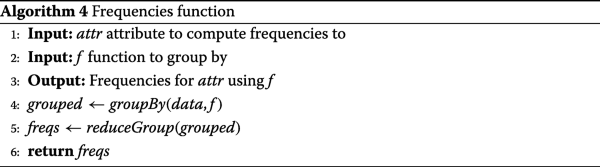Algorithm 5 shows the pseudocode for OFS , this algorithm maps each label and feature with their corresponding value for the original OFS algorithm.Algorithm 6 shows pseudocode for IDA . This algorithm first computes the cut points for the dataset with the desired number of bins. In order to compute the cut points, each instance is mapped to the result of IDA, which returns the computed cut points. To do so, each feature is zipped with its index, and then folded with its corresponding class label and a zero feature vector that will be filled in each iteration of the fold operation, with the returned value of the IDA algorithm. Once cut points are stored, line 5 in Algorithm 6 discretizes the data according to those cut points.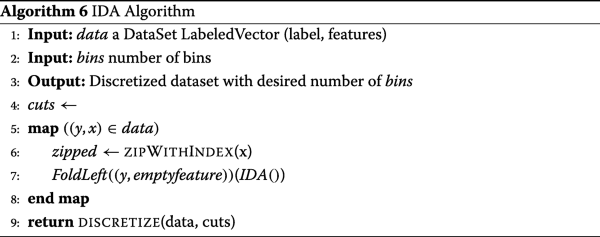Algorithm 7 shows pseudocode for PiD , this algorithm first initializes the required data structures using a map function, this map function expands the dataset and adds it to a histogram and a count of the total number of instances seen so far. Then this data is reduced, computing in each reduce step the layers one and two as described in the original algorithm . Once this reduce stage has been completed, it returns the discretized data using the previously computed cut points.Algorithm 8 shows pseudocode for LOFD . This algorithm first instantiates a LOFD helper, and maps the data according to the computed cut points this helper returns. Once all cutpoints have been collected, the reduce function extracts only the most recently computed cut points and applies discretization based on those same cut points.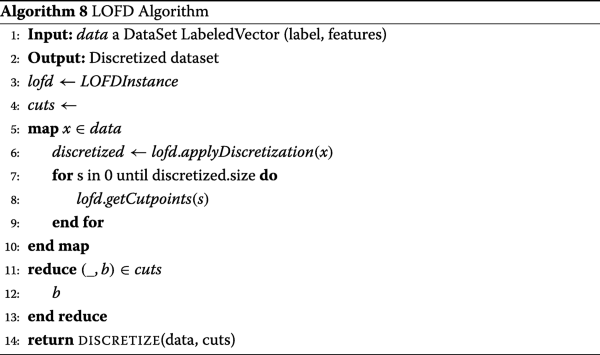## Results

This section presents in detail the six algorithms implemented in Apache Flink, there are three algorithms for feature selection and three for discretization.

### Examples

The software has been implemented in the Scala programming languageFootnote 2. As mentioned above, DPASF consists of six algorithms for data streams, three discretization methods and three feature selection methods. The software can be found on GitHubFootnote 3. The next section presents how to use each algorithm in Apache Flink.

### Usage

#### Feature Selection

##### FCBF

In order to benefit from the Apache Flink framework, symmetrical uncertainty computations for each pair of attributes are distributed across each node in order to speed up the process.

Suppose the data set to be used is the Abalone DataSetFootnote 4, load it into Apache Flink: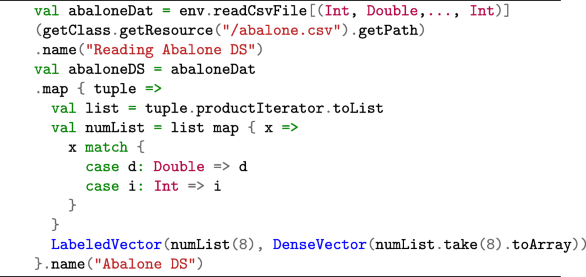Then, a FCBFTransformer must be instantiated, configure its parameters and finally define a pipeline: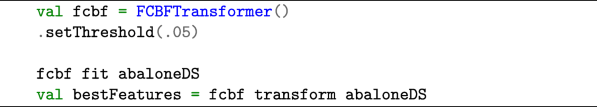After fitting the algorithm, calling transform on fcbf will return the Abalone data set with the most important features.

##### InfoGain

For this algorithm, each attribute’s Information Gain value is computed in parallel.

The use of InfoGainTransformer is similar: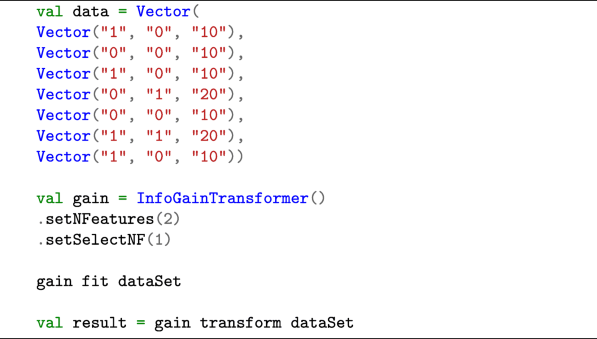##### OFS

One difference of OFS with respect to the previous algorithms is that it does not require a fitting phase:#### Discretization

In this section the usage of the discretization methods is presented. The IrisFootnote 5 DataSet was used, loaded as: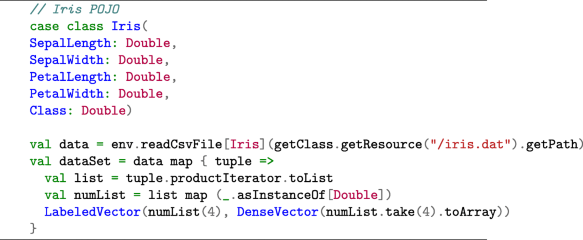##### IDA

For IDA, cut points are computed in parallel, in order to get the most recent computed cut point, data is reduced to get the latest set of cuts.##### PiD

For PiD, the histogram required is shared across all nodes. After the histogram is initialized, data is reduced in order to produce the final histogram, where the cut points required to perform the discretization are found.

In PiD, data must be normalized in the previous step, so a ChainTransformer is used in the pipeline.##### LOFD

For LOFD, a PiD-like approach is used. First, all features are mapped in order to extract the necessary information from them, then, data is reduced to extract the final cut points to perform discretization.### Results

The experimental set up has used two datasets, ht_sensor and skin_nonskin, and are described in Table 1.

All algorithms have been tested with KNN and Decision Trees using 5-fold cross validation. A baseline is fitted without any preprocessing steps, and another is fitted with the corresponding preprocessing algorithm. In addiction KNN has been fitted with k = 3 and k = 5.

All the feature selection methods have been set up to select 50% of the features.

Table 2 shows the amount of time it took to preprocess the data. The worst algorithm by far is IDA, which took about 5 h to finish for ht_sensor. On the contrary, the fastest was InfoGain. OFS could not be measured as it only accepts binary datasets. It is worth mentioning that these experiments could not have been possible in normal environments due to the amount of time they would have taken.

For all experiments we have used a cluster composed of 14 computing nodes. The nodes hold the following characteristics: 2 x Intel Core i7-4930K, 6 cores each, 3.40 GHz, 12 MB cache, 4 TB HDD, 64 GB RAM. Regarding software, we have used the following configuration: Apache Flink 1.6.0, 238 TaskManagers (17 TaskManagers/core), 49 GB RAM/node.

Tables 3 and 4 show the accuracy obtained by the algoritms, as well as the accuracy without any preprocessing. The three feature selection methods obtain considerable results, even when they are configured to remove half the features on the datasets. FCBF is not among the best, but it is among the fastest. This may be due to the fact that it avoids computing pairwise comparisons when selecting features. Also, FCBF can not be set to select a fixed number of features, it uses a threshold to select features based on its SU value, so in some cases it will select less than half the features. InfoGain also gives excellent results, close to the baseline and even improves when k=3. Among the discretizers, PiD outperforms baseline in all cases except for skin-nonskin with k=3.

Table 5 shows accuracy for a Decision Tree model, results are consistent with the previous model. In general, feature selection methods result in a decrease in accuracy whereas discretization methods are consistent with baseline, albeit PiD improves accuracy.

## Conclusions

In this paper we have tackled the Big Data stream preprocessing problem. We have created a library for Big Data data stream preprocessing called DPASF that is implemented in the Big Data streaming framework Apache Flink. This library includes six popular data preprocessing algorithms. Three of the algorithms are focused on discretization, while the other three are focused on feature selection tasks. All the algorithms have been redesigned for the map reduce framework so they can cope with Big Data datasets.

The performance of the six algorithms in Big Data scenarios has been analyzed using two Big Data datasets. Experimental results have shown that preprocessing can improve the original accuracy in a short amount of time. We have also observed that choosing the right technique is crucial depending on the problem and the classifier used.

## Availability and requirements

Project name: DPASF

Operating system(s): Platform independent

Programming language: Scala

## Availability of data and materials

ht_sensor and skin_nonskin datasets are freely available in .

## Abbreviations

DPASF:

Data preprocessing algorithms for streaming in Flink

FCBF:

Fast correlation-based filter

IDA:

Incremental discretization algorithm

InfoGain:

Information gain

LOFD:

Local online fusion discretizer

OFS:

Online feature selection

PiD:

Partition incremental discretization algorithm

## References

1. García S, Ramírez-Gallego S, Luengo J, Benítez JM, Herrera F. Big data preprocessing: methods and prospects. Big Data Analytics. 2016; 1(1):9.

2. Saeed F. Towards quantifying psychiatric diagnosis using machine learning algorithms and big fmri data. Big Data Analytics. 2018; 3(1):7.

3. García S, Luengo J, Herrera F. Tutorial on practical tips of the most influential data preprocessing algorithms in data mining. Knowledge-Based Systems. 2016; 98:1–29.

4. García S, Luengo J, Herrera F. Data Preprocessing in Data Mining. University of Granada: Springer; 2015.

5. García-Gil D, Luengo J, García S, Herrera F. Enabling Smart Data: Noise filtering in Big Data classification. Information Sciences. 2019; 479:135–152.

6. Ramírez-Gallego S, Krawczyk B, García S, Woźniak M, Herrera F. A survey on data preprocessing for data stream mining: Current status and future directions. Neurocomputing. 2017; 239:39–57.

7. Spark A. Apache Spark: lightning-fast cluster computing. http://spark.apache.org.

9. Friedman B. Introduction to Apache Flink : Stream Processing for Real Time and Beyond. Sebastopol, CA: O’Reilly Media; 2016.

10. García-Gil D, Ramírez-Gallego S, García S, Herrera F. A comparison on scalability for batch big data processing on apache spark and apache flink. Big Data Analytics. 2017; 2(1):1.

11. Carbone P, Katsifodimos A, Ewen S, Markl V, Haridi S, Tzoumas K. Apache flink : Stream and batch processing in a single engine. Bulletin of the IEEE Computer Society Technical Committee on Data Engineering. 2015; 36(4):28–38. QC 20161222.

12. Katakis I, Tsoumakas G, Vlahavas I. On the utility of incremental feature selection for the classification of textual data streams In: Bozanis P, Houstis EN, editors. Advances in Informatics. Berlin, Heidelberg: Springer: 2005. p. 338–348.

13. Yu L, Liu H. Feature selection for high-dimensional data: A fast correlation-based filter solution.2003. p. 856–863.

14. Wang J, Zhao P, Hoi SCH, Jin R. Online feature selection and its applications. IEEE Transactions on Knowledge and Data Engineering. 2014; 26(3):698–710.

15. Webb GI. Contrary to popular belief incremental discretization can be sound, computationally efficient and extremely useful for streaming data. In: Proceedings of the 2014 IEEE International Conference on Data Mining. ICDM ’14. Washington, DC: IEEE Computer Society: 2014. p. 1031–1036. URL https://doi.org/10.1109/ICDM.2014.123.

16. Ramírez-Gallego S, García S, Herrera F. Online entropy-based discretization for data streaming classification. Future Generation Computer Systems. 2018; 86:59–70.

17. Pinto C. Discretization from data streams: applications to histograms and data mining. In: In Proceedings of the 2006 ACM Symposium on Applied Computing (SAC’06: 2006. p. 662–667.

18. Alcalde A. elbaulp/DPASF: 0.1.1 release. 2018. https://doi.org/10.5281/zenodo.1451506.

19. Landset S, Khoshgoftaar TM, Richter AN, Hasanin T. A survey of open source tools for machine learning with big data in the hadoop ecosystem. Journal of Big Data. 2015; 2(1):24.

20. Rao TR, Mitra P, Bhatt R, Goswami A. The big data system, components, tools, and technologies: a survey. Knowledge and Information Systems. 2018. https://doi.org/10.1007/s10115-018-1248-0.

21. Ramírez-Gallego S, Fernández A, García S, Chen M, Herrera F. Big Data: Tutorial and guidelines on information and process fusion for analytics algorithms with MapReduce. Information Fusion. 2018; 42:51–61.

22. García-Gil D, Ramírez-Gallego S, García S, Herrera F. Principal Components Analysis Random Discretization Ensemble for Big Data. Knowledge-Based Systems. 2018; 150:166–174.

24. Laney D. 3D Data Management: Controlling Data Volume, Velocity, and Variety: META Group; 2001. https://www.bibsonomy.org/bibtex/263868097d6e1998de3d88fcbb7670ca6/sb3000.

25. Gama J. Learning from Data Streams : Processing Techniques in Sensor Networks. Berlin New York: Springer; 2007.

26. Gama Ja, žliobaitė Ie, Bifet A, Pechenizkiy M, Bouchachia A. A survey on concept drift adaptation. ACM Comput Surv. 2014; 46(4):44–14437.

27. Li J, Cheng K, Wang S, Morstatter F, Trevino RP, Tang J, Liu H. Feature selection: A data perspective. ACM Computing Surveys (CSUR). 2017; 50(6):94.

28. Ramírez-Gallego S, Mouriño-Talín H, Martínez-Rego D, Bolón-Canedo V, Benítez J. M, Alonso-Betanzos A, Herrera F. An information theory-based feature selection framework for big data under apache spark. IEEE Transactions on Systems, Man, and Cybernetics: Systems. 2018; 48(9):1441–1453.

29. Press WH, Teukolsky SA, Vetterling WT, Flannery BP. Numerical recipes in c. Cambridge University Press. 1988; 1:3.

30. Ramírez-Gallego S, García S, Mouriño-Talín H, Martínez-Rego D, Bolón-Canedo V, Alonso-Betanzos A, Benítez JM, Herrera F. Data discretization: taxonomy and big data challenge. Wiley Interdisciplinary Reviews: Data Mining and Knowledge Discovery. 2016; 6(1):5–21.

31. Vitter JS. Random sampling with a reservoir. ACM Trans Math Softw. 1985; 11(1):37–57.

32. Wood D, Informatica V, H BC, Leeuwen JV, Leeuwen JV. Interval heaps. The Computer Journal. 1987; 36:209–216.

33. Fayyad UM, Irani KB. On the handling of continuous-valued attributes in decision tree generation. Machine Learning. 1992; 8(1):87–102.

34. Fayyad U, Irani K. Multi-interval discretization of continuous-valued attributes for classification learning. 1993.

35. Witten IH, Frank E, Hall MA, Pal CJ. Data Mining : Practical Machine Learning Tools and Techniques. Cambridge, MA: Morgan Kaufmann Publisher; 2017.

36. Zighed DA, Rabaséda S, Rakotomalala R. FUSINTER: A method for discretization of continuous attributes. International Journal of Uncertainty, Fuzziness and Knowledge-Based Systems. 1998; 06(03):307–326.

37. Dheeru D, Karra Taniskidou E. UCI Machine Learning Repository. 2017. http://archive.ics.uci.edu/ml.

Not applicable.

## Funding

This work is supported by the Project BigDaP-TOOLS - Ayudas Fundación BBVA a Equipos de Investigación Científica 2016.

## Author information

Authors

### Contributions

AA carried out the implementation of the library and drafted the manuscript. DG helped with the implementation and draft of the manuscript. SG and FH conceived the library, participated in its design and coordination and helped to draft the manuscript. All authors read and approved the final manuscript.

### Corresponding authors

Correspondence to Alejandro Alcalde-Barros or Diego García-Gil.

## Ethics declarations

Not applicable.

Not applicable.

### Competing interests

The authors declare that they have no competing interests.